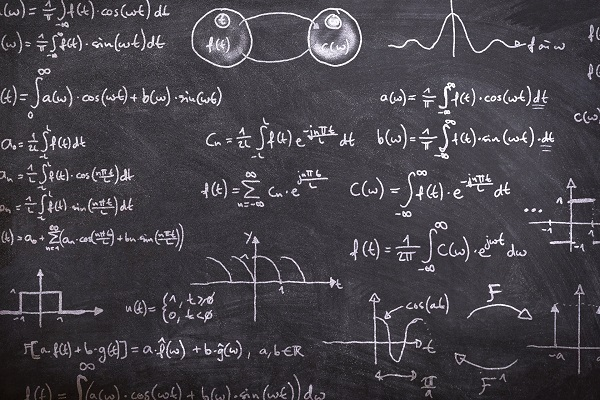• #### Degree Finder

Before you jump into earning a master’s in petroleum engineering – one of the highest-paying master’s degrees – you should know what you’re getting into. Petroleum engineering and other disciplines of engineering may not be math occupations specifically, but they do require you to have a strong background in mathematics.

## Math Coursework in Petroleum Engineering ProgramsIMAGE SOURCE: Pixabay, public domain

## Featured Programs

How extensive your formal math coursework will be in a petroleum engineering program depends on the level of your program. Graduate degree programs in petroleum engineering may not include any math coursework because applicants are expected to already have competence in mathematics. Master’s degree programs in this field may have a list of math prerequisites that applicants must have already completed before enrolling in the program.

Undergraduate petroleum engineering programs are likely to include at least two college-level calculus courses, a class in differential equations and a statistics course. Calculus is the mathematical study of modeling and calculating change. Generally, you can expect a calculus I course to serve as your introduction to calculus, covering the differentiation, integration and – in a limited capacity – application of functions pertaining to just one variable. Calculus II courses progress to multivariable functions and may include studies of vectors, vector fields, vector-valued functions, line integrals and surface integrals.

The two math courses that ABET (the Accreditation Board for Engineering and Technology) requires accredited petroleum engineering programs to have are differential equations and probability and statistics. Like calculus, a course in differential equations focuses on the mathematical ways of calculating change. What distinguishes differential equations from other areas of mathematics is that solving a math problem doesn’t mean figuring out an unknown number in an equation but instead using information about a derivative of a function to solve for the unknown function.

Finally, statistics is a mathematical science that revolves around gathering, analyzing, manipulating and interpreting quantitative data. Generally, statistics isn’t about solving for a variable but rather drawing conclusions from empirical data. A major topic in the study of statistics is probability, which refers to uncertainty and the likelihood of an event or outcome occurring due to random chance.

Math is a subject many people find a little bit daunting. The earlier petroleum engineers begin learning the relevant math disciplines, the better. The United States Bureau of Labor Statistics recommends taking algebra, calculus and trigonometry in high school.

## The Role of Math in Engineering

The principles and practices of engineering design are based in math and the natural sciences. As such, several courses specific to engineering are heavily based in mathematics. In ABET-accredited programs, the engineering course topics through which petroleum engineering students must continue to build their mathematics knowledge are thermodynamics, fluid mechanics and the strength of materials.

Thermodynamics is most simply described as the study of heat and mechanics, or the movement of heat between objects. The study of thermodynamics generally requires a good foundation in calculus. Fluid mechanics refers to the study of fluid behavior, motion and response to the application of physical forces. Again, a base knowledge in calculus is important for grasping this branch of physical science, as is some background in differential equations. Finally, coursework in the strength of engineering materials may require you to calculate forces, such as tension and compression, and how they affect different materials used in engineering. Having some knowledge of differential equations can help you perform these calculations.

What’s important to know is that the math used in engineering is generally applied math, not theoretical or abstract math. Rather than looking to advance pure knowledge of the field of mathematics, you’re using mathematical principles to build solutions.

## How Petroleum Engineers Use Mathematics

How exactly does all of this math coursework pertain to your future career as a petroleum engineer? Whether they’re designing the structures for oil rigs and water management systems, developing safe procedures for drilling and well completion or coming up with cost-effective options for reservoir management and resource extraction, petroleum engineers are always guided by mathematical and scientific principles. They don’t build prototypes based on hunches. Instead, they perform calculations – personally or with the use of computer software – to design systems, processes, structures and machines that meet the needs of the gas and oil industry and then use software to analyze, model and run simulations on their design ideas.

As a petroleum engineer, you won’t sit at a desk all day solving math equations for the pure purpose of doing math. Math disciplines like calculus, differential equations and statistics are some of the tools you will use to solve problems in the petroleum industry.